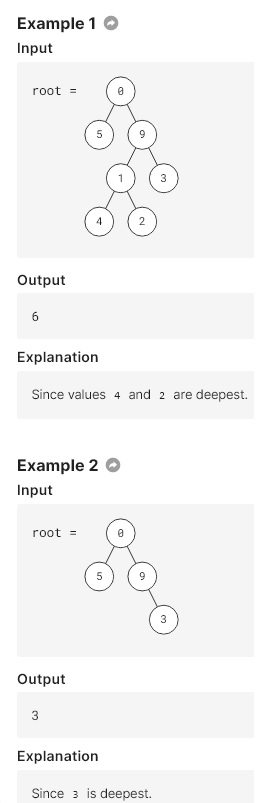# GoLang: Breadth First Search Algorithm to Compute the Deepest Leaves Sum of Binary Tree

in blurt •  7 days ago
Given a binary tree root, find the sum of the deepest node values.

Constraints
n ≤ 100,000 where n is the number of nodes in rootHint:
You need to get the sum of all the nodes at the last level of the tree. How do you traverse level by level?

### GoLang: Deepest Leaves Sum via Breadth First Search

There is no inbuilt Queue object in GoLang, but we can use array/list to achieve the same task. To enque, we just need to use append method. To deque, we can use the array slicing e.g. arr[1:]. To peek the front of the queue, we can just get the value of the first element e.g. arr.

To compute the sum of the deepest leaves, we can use the Breadth First Search Algorithm. Let's keep updating the sum of the current level when we expand the nodes during BFS.

```/**
* Definition for a binary tree node.
* type TreeNode struct {
*     Val int
*     Left *TreeNode
*     Right *TreeNode
* }
*/
func deepestLeavesSum(root *TreeNode) int {
if root == nil {
return 0
}
var Q = make([]*TreeNode, 0)
Q = append(Q, root)
var curSum int
for len(Q) > 0 {
var sz = len(Q)
curSum = 0
for i := 0; i < sz; i ++ {
curSum += Q[i].Val
if Q[i].Left != nil {
Q = append(Q, Q[i].Left)
}
if Q[i].Right != nil {
Q = append(Q, Q[i].Right)
}
}
Q = Q[sz:]
}
return curSum
}
```

The time complexity is O(N) and so is space complexity where N is the number of the nodes in the given binary tree as we need to visit each node exactly once.

See posts of computing the sum of the deepest leaves for a given binary tree:

Reposted to Blog

## NEW! Following my Trail (Upvote or/and Downvote)

Follow me for topics of Algorithms, Blockchain and Cloud.
I am @justyy - a Steem Witness
https://steemyy.com

#### Delegation Service

• Delegate 1000 to justyy: Link
• Delegate 5000 to justyy: Link
• Delegate 10000 to justyy: Link

#### Support me

If you like my work, please:

1. Delegate SP: https://steemyy.com/sp-delegate-form/?delegatee=justyy
2. Vote @justyy as Witness: https://steemyy.com/witness-voting/?witness=justyy&action=approve
3. Set @justyy as Proxy: https://steemyy.com/witness-voting/?witness=justyy&action=proxy
Alternatively, you can vote witness or set proxy here: https://steemit.com/~witnesses

Sort Order:
·  7 days ago

Congratulations! This post has been upvoted by the @blurtcurator communal account,
You can request a vote every 12 hours from the #getupvote channel in the official Blurt Discord.Don't wait to join ,lots of good stuff happening there.

·  4 days ago

Congratulations, your post has been upvoted by @r2cornell, which is the curating account for @R2cornell's Discord Community.

Curated by <@bestkizito >